# [Paper-ASR][中文笔记] A Comparative Study on Neural Architectures and Training Methods for Japanese Speech Recognition

Published:

Last Updated: 2021-07-04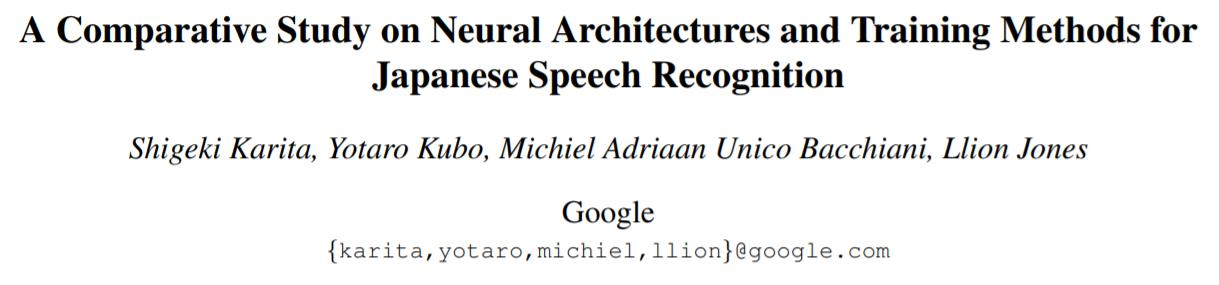## 神经网络架构

### Conformer Encoder

Conformer Encoder和 BLSTM Encoder 的区别就是用 Conformer 模块代替了 BLSTM 层。Figure 1为Conformer模块的示意图。Conformer使用基于相对位置编码 (Relative Positional Encoding) 的多头注意力机制建模全局特征，对于长度为 T 的输入序列，多头自注意力的计算和内存复杂度都是 O(T^2)。

Figure 2 展开了 Figure 1 中的 Convolution Module，该模块用于建模局部特征。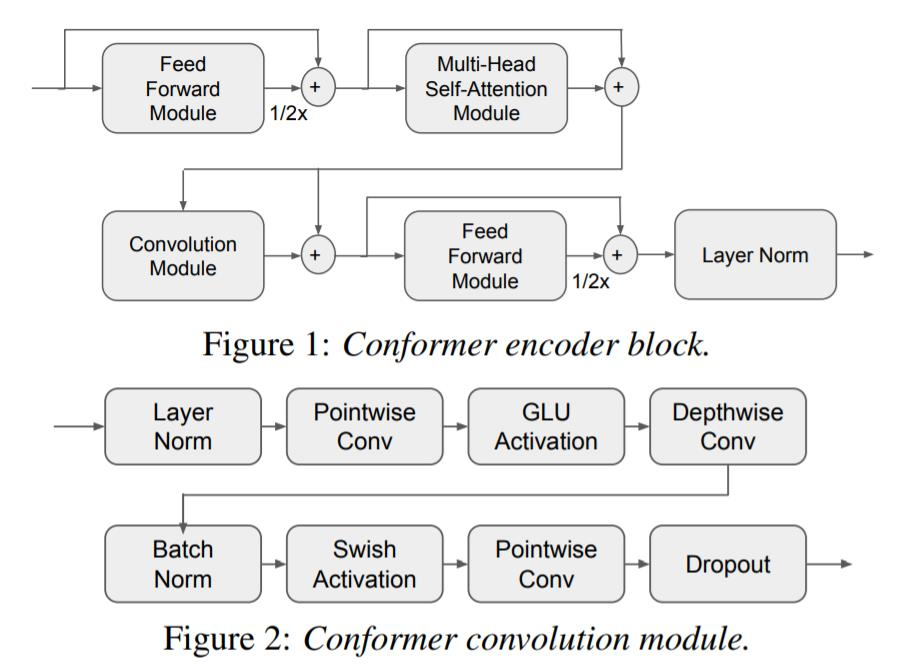Conformer可以被并行化训练，因此相比 BLSTM 训练速度会快很多；但是缺点就是上述的注意力复杂度，当输入序列很长的时候可能会导致性能瓶颈。在本文实验中用 training throughput 评估。

### CTC Decoder

CTC Decoder就是一层线性层 + Softmax 激活函数，CTC 通过引入一个表示 Encoder 编码的特征 X 和输出序列 Y 之间的 Alignment 的变量 Z 来预测输出序列的分布。Y 和 Z 之间的关系由一个多对一的映射 B 给出：

$Y = B(Z)$

B(Z) 就是将 Z 中的 blank 和 重复 symbol 去掉，举个栗子：B(aa<blank>b) = ab。并且， Z 中的每一个 symbol $Z_t$ 被预测到的概率都是对应 X 中的一帧特征经过 CTC Decoder 转换预测得到的概率分布中的第 $Z_t$ 个元素，即$C_t=\text{CTC}(X_t)$ ， $P(Z_t) = C_{t, Z_t}$ 。从这里我们也可以看出来，CTC进行了条件独立性假设，即 $Z_t$ 的预测与上下文 $Z_{t-1}$ 和 $Z_{t+1}$ 均无关。

$p_{ctc}(B(Z)=Y|X)=\prod_{t=1}^T C_{t, Z_t}$ $L_{ctc} = - log \sum_{Z \in B^{-1}(Y)} p_{ctc}(B(Z) = Y | X)$

### Transducer Decoder

Transducer 和 CTC 原理类似，都是通过最大化可能的 Alignment 概率进行训练，区别在于 Transducer 去掉了CTC的条件独立性假设，而是递归地建模条件分布。

$L_{\text{transducer}} = - log \sum_{Z \in B^{-1}(Y)} \prod_t p_\text{transducer} (Z_t | X, B(Z_{1:t-1}))$

Transducer Decoder 一般采用循环神经网络（RNN）进行递归式的编码。

### Attention Decoder

Attention Decoder 一般由两个模块组成：注意力模块 Attend() 和 输出模块 Spell()。不像 CTC 和 Transducer，它不需要显式地对齐每一个语音帧和输出符号。

$L_\text{att} = - log \prod_t p_\text{att} (Y_t | X, Y_{1:t-1})$

Attention Decoder 在 NLP 的各种序列生成任务（Seq2Seq）中有着广泛的运用，这里就不详细介绍了。

## 训练技巧

### SpecAugment

def spec_augmentation(x,
warp_for_time=False,
max_t=50,
max_f=10,
max_w=80):
""" Deep copy x and do spec augmentation then return it
Args:
x: input feature, T * F 2D
max_t: max width of time mask
max_f: max width of freq mask
max_w: max width of time warp
Returns:
augmented feature
"""
y = np.copy(x)
max_frames = y.shape
max_freq = y.shape

# time warp
if warp_for_time and max_frames > max_w * 2:
center = random.randrange(max_w, max_frames - max_w)
warped = random.randrange(center - max_w, center + max_w) + 1

left = Image.fromarray(x[:center]).resize((max_freq, warped), BICUBIC)
right = Image.fromarray(x[center:]).resize(
(max_freq, max_frames - warped), BICUBIC)
y = np.concatenate((left, right), 0)
start = random.randint(0, max_frames - 1)
length = random.randint(1, max_t)
end = min(max_frames, start + length)
y[start:end, :] = 0
start = random.randint(0, max_freq - 1)
length = random.randint(1, max_f)
end = min(max_freq, start + length)
y[:, start:end] = 0
return y


### Exponential Moving Average (EMA)

EMA 通过维护一个影子权重来提高模型训练的稳定性和泛化能力，其基本假设是，模型权重在最后的n步内，会在实际的最优点处抖动，所以我们取最后n步的平均，能使得模型更加的鲁棒。每一个训练 step $k$ 后，EMA 的模型参数 $\theta_k’$ 通过下面的公式计算：

$\theta_k' = \gamma \theta_{k-1}' + (1 - \gamma) \theta_k$

class ExponentialMovingAverage(object):
def __init__(self, model, decay=0.9999):
self.model = model
self.decay = decay
self.backup = {}

for name, param in ema_model.named_parameters():

def register(self):
for name, param in self.model.named_parameters():

def update(self):
for name, param in self.model.named_parameters():
new_average = (1.0 - self.decay) * param.data + self.decay * self.shadow[name]

# 以下函数用于评估模型时的参数变换
for name, param in self.model.named_parameters():
self.backup[name] = param.data

def restore(self):
for name, param in self.model.named_parameters():
assert name in self.backup
param.data = self.backup[name]
self.backup = {}


### Variational Noise

Variational Noise 也能提高神经网络的泛化能力，具体做法是对原参数加上服从正态分布的噪声样本，得到带噪参数用于训练。（本文只将其用于 embedding 和 LSTM 层）

$\theta' = \theta + n, n \sim \text{Normal}(0, \sigma^2)$

## 实验结果

### 模型架构 对比

Encoder 对于 CER 结果的影响明显大于 Decoder。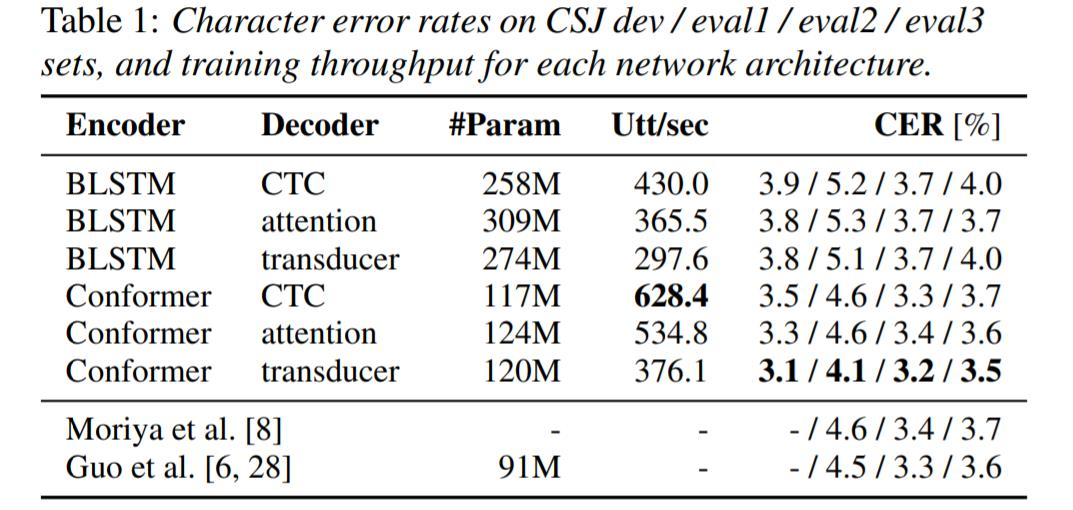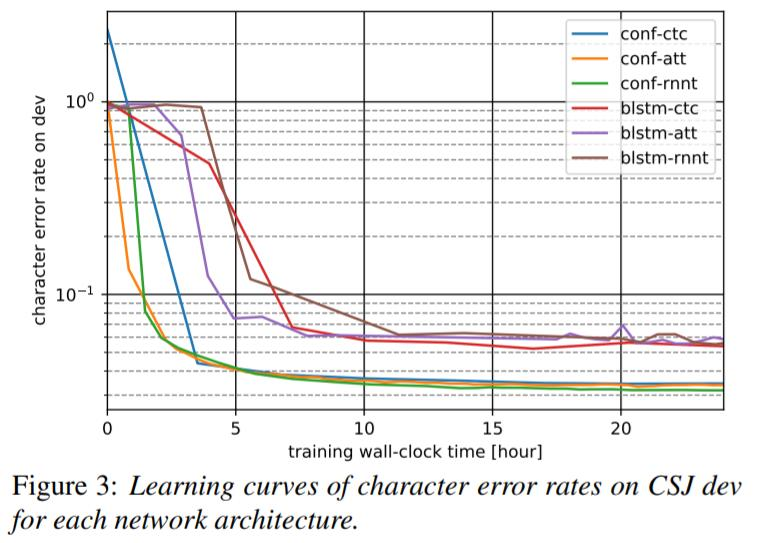### 训练技巧 对比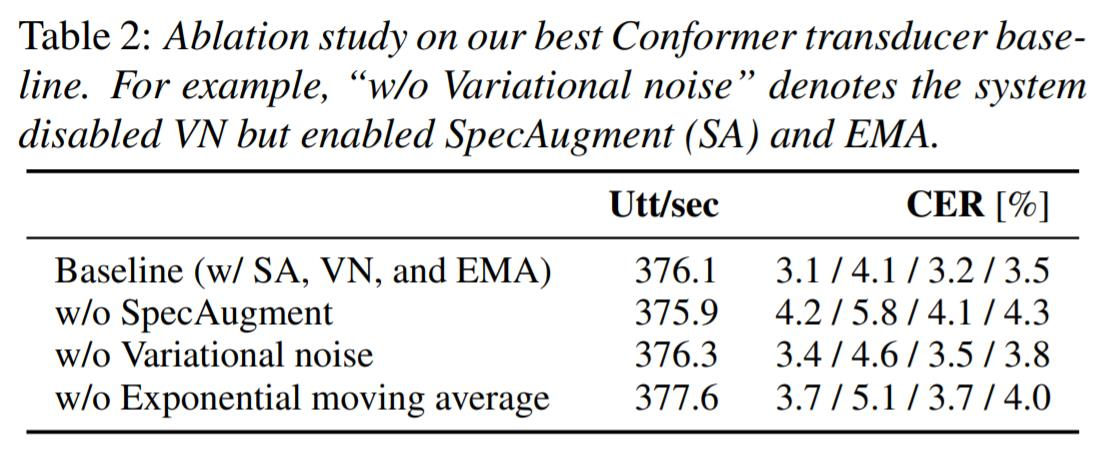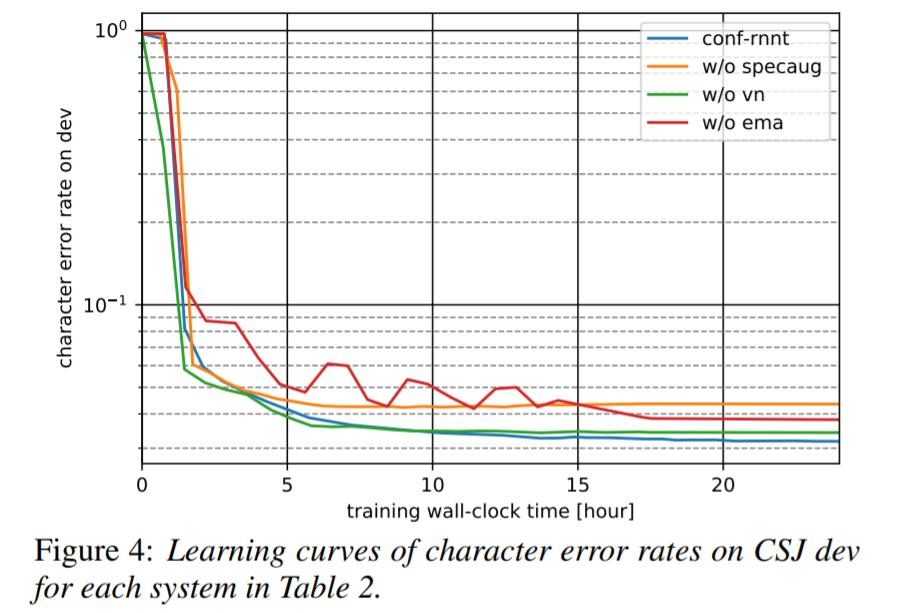### 计算复杂度 对比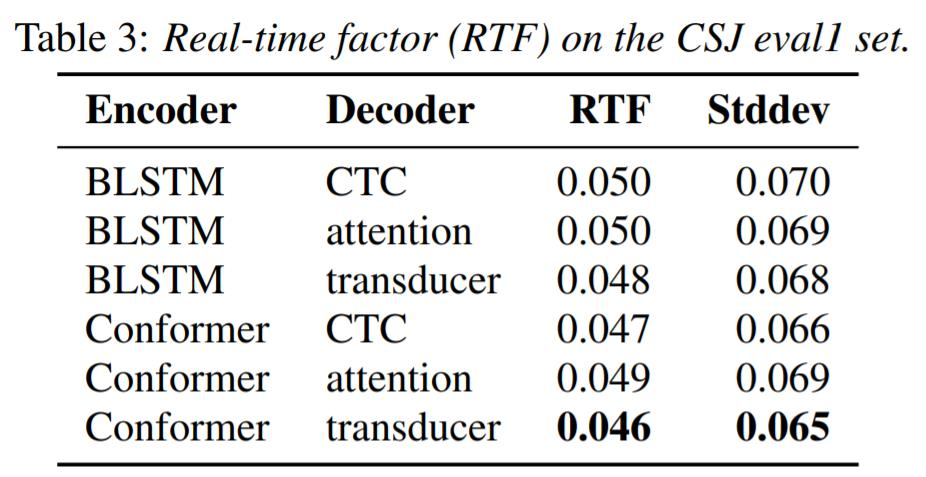Tags: